Next: Proof of Covariance Up: Dirac Equation Previous: Free Motion of a

# Covariant Form of the Dirac Equation

We now cast the Dirac equation into a more apparent covariant form. Multiplying (5.3) by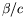and defining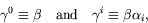(5.96)

where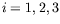, we have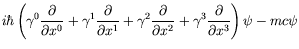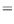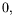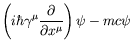(5.97)

where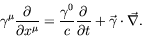(5.98)

In terms of the momentum operator we write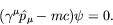(5.99)

Introducing the Feynman dagger, or slash notation, for 4-vector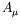, we have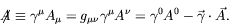(5.100)

Also notice that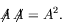(5.101)

We write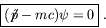(5.102)

We introduce the electromagnetic interaction by the usual minimal substitution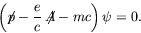(5.103)

Let us study the properties of the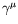matrices.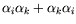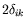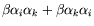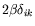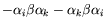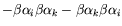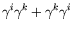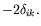(5.104)

And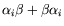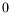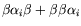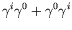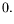(5.105)

Since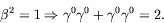(5.106)

Therefore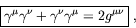(5.107)

where the matrices are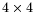and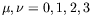. Although the Dirac matricesare written with Greek indices, they are not four vectors. Rather, they have the same value in every frame.

The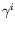matrices are anti-hermitian and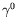is hermitian: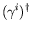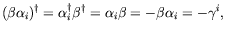(5.108)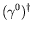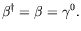(5.109)

This can be summarized by writing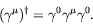(5.110)

Using our previous representation (equation 5.10),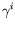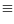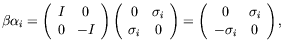(5.111)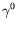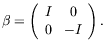(5.112)Next: Proof of Covariance Up: Dirac Equation Previous: Free Motion of a
Douglas M. Gingrich (gingrich@ ualberta.ca)
2004-03-18